# Consecutive Numbers Questions for Competitive Exams

Sum of consecutive numbers series (odd, even) for Competitive Exams. Online practice test in Hindi and English based on the previous questions of SSC Exams. Sum of consecutive numbers is useful concept in the areas of mathematics, including algebra, geometry, and calculus. It is used to find the total of a series of numbers or to solve equations involving consecutive numbers.

## Number System Type 6 : Sum of Consecutive Numbers

Question 1:
The sum of all the natural numbers from 51 to 100 is
51 से 100 तक की सभी प्राकृत संख्याओं का योग है
(1) 5050
(2) 4275
(3) 4025
(4) 3775

(4) 3775
We have, 1 + 2 + 3 …….. +n =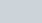$\frac{n.(n+1)}{2}$
= 51 + 52 + ….. + 100 = (1 + 2 + ……. 100) – (1 + 2 + … + 50)
=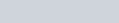$\frac {(100)(101)}{2} - \frac {(50)(51)}{2}$
= 5050 – 1275 = 3775

Question 2:
The sum of all the 3-digit numbers, each of which on division by 5 leaves remainder 3, is
सभी 3-अंकीय संख्याओं का योग, जिनमें से प्रत्येक को 5 से विभाजित करने पर शेष 3 रहता है, है
(1) 180
(2) 1550
(3) 6995
(4) 99090

(4)99090
According to the question, First number =a = 103 Last number = l = 998
If the number of such numbers ben, then,
998 = 103 + (n – 1) × 5
(n – 1) × 5= 998 – 103 = 895
n – 1 =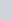$\frac{89}{5}$= 179
= n = 180
S =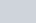$\frac{n(a+l)}{2}$
=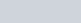$\frac{(180) (103+998)}{2}$
= 90 × 1101 = 99090

Question 3:
Out of six consecutive natural numbers, if the sum of first three is 27, what is the sum of the other three ?
छह लगातार प्राकृतिक संख्याओं में से, यदि पहले तीन का योग 27 है, तो अन्य तीन का योग क्या है?
(1) 36
(2) 35
(3) 25
(4) 24

(1) 36
x +x + 1 +x+ 2 = 27
3x + 3 = 27
3x = 24
x = 8
Three consecutive no’s whose sum is 27 are 8, 9,10. Hence, next 3 consecutive no’s having 36 as sum are 11, 12 and 13

Question 4:
What is the arithmetic mean of first 20 odd natural numbers ?
प्रथम 20 विषम प्राकृतिक संख्याओं का अंकगणितीय माध्य क्या है?
(1) 19
(2) 17
(3) 22
(4) 20

(4) 20
Sum of first n odd natural numbers
=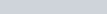$n^2 = 20^2 = 400$
Required average =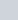$\frac{400}{ 20}$= 20

Question 5:
The sum of three consecutive natural numbers each divisible by 5, is 225. The largest among them is
तीन क्रमागत प्राकृत संख्याओं का योग, जिनमें से प्रत्येक 5 से विभाज्य है, 225 है। उनमें से सबसे बड़ी संख्या है
(1) 85
(2) 75
(3) 70
(4) 80

x =$\frac{240} {3}$ = 80